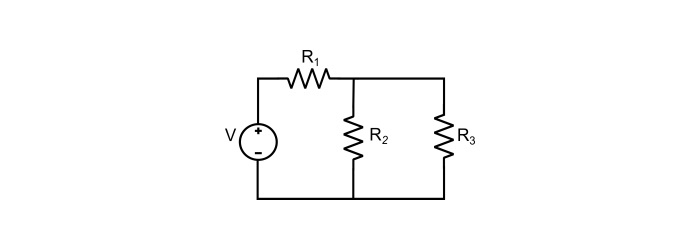# Characteristics of Network and Electric Circuit

## Electric Network

An electrical network is an interconnection of electrical components (like sources, resistors, inductors, capacitors, diodes, transistors etc.). An electrical network need not contain a closed path for the flow of electric current. Some examples of the network are shown in the figure given below.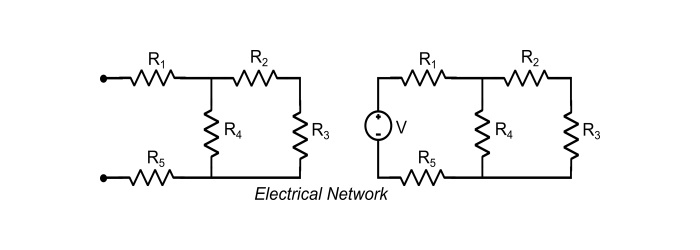## Characteristics of Electric Network

An electric network possesses the following characteristics −

• It is an interconnection of electrical components.

• Electric network need not provide a return path for the current.

• It may or may not contain active electrical components.

• It may be consist of, passive elements, active elements or both active and passive elements.

• Examples of electric network are − Resistive network, inductive network, capacitive network, diode network, etc.

## Types of Electric Networks

• Passive Network − A passive network does not contain any sources of energy. They consist only passive circuit elements like resistors, inductors and capacitors etc.

• Active Network − An active network contains one or more sources of energy (like voltage source or current source), they can supply energy to the network indefinitely. An active network is also termed as electric circuit.

• Linear Network − A network is said to be linear, if it consists of the circuit elements that obeys the principle of homogeneity and superposition. Resistive network is an example of linear network.

• Non–Linear Network − All the network that are not linear i.e. do not obey the homogeneity and superposition principle are called no–linear networks. The network consisting of iron cored inductor is an example of non-linear network.

• Lumped Network − These networks consist of elements whose physical dimensions are very small as compared to the wavelength of quantity. The network is called as lumped network. Examples − Resistor, Inductor and Capacitor.

• Distributed Network − When the physical dimensions of the elements of the network are comparable with the wavelength of the electrical quantity. The network is said to be a distributed network. Transmission lines are the examples of the distributed network.

## Electric Circuit

An electric circuit is, an electric network that contains a closed path for the flow of electric current. It provide a return path for the current to flow in the circuit. In other words, a network that contains active electrical components is known as an electric circuit. The circuit elements in an electric circuit are connected in series connection, parallel connection or series-parallel connection. Hence, an electric circuit is a network, but the converse need not be true.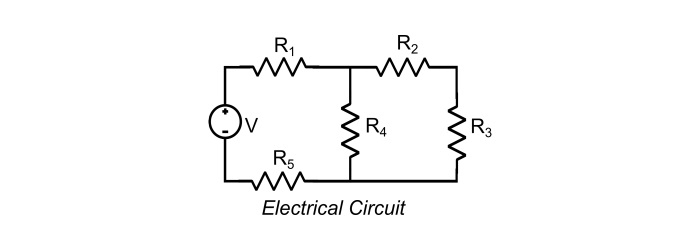## Characteristics of Electric Circuit

An electric circuit possesses the following characteristics −

• Electric circuit is also an interconnection of electrical components, i.e. a circuit is also an electric network.

• In an electric circuit, there must be a return path to for a closed loop for the current to flow.

• It must contain at least one source of energy i.e. active circuit element need to be present in the circuit.

• An electric circuit is the combination of both active and passive elements that are connected in series, parallel or series-parallel connections.

• In an electric circuit, flow of electrons takes place from negative terminal to positive terminal of the source.

• The direction of flow of conventional electric current is from positive to negative terminal of the source.

• Examples of electric circuits are − resistive circuit, inductive circuit, capacitive circuit, electronic circuit etc.

## Types of Electric Circuits

• Open Circuit − If there is a no current flow in the circuit due to any disconnection, the circuit is called an open circuit.• Closed Circuit − If there is no discontinuity in the circuit and current can flow in the circuit, the circuit is called closed circuit.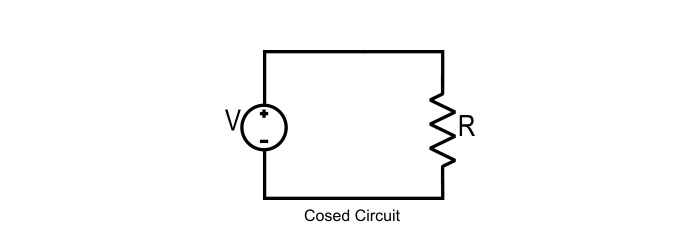• Short Circuit − In a DC system, when the positive terminal and negative terminal connected to each other through a zero resistance path, it is termed as short circuit. In case of AC system, if phase and neutral touches each other or any phase touches the ground or two phases touches each other, then these conditions are also termed as short circuit.• Series Circuit − When circuit elements are connected one after another as the second end of one element joined to the first end of the another element, so that, there is only one path for the flow of current is available, the resulting circuit is known as series circuit.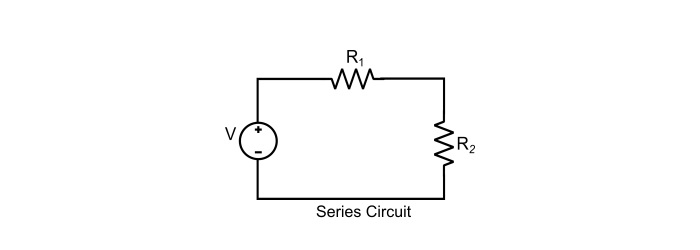• Parallel Circuit − If the circuit elements are connected in such manner that the voltage across each element is same, the resulting circuit is called as parallel circuit.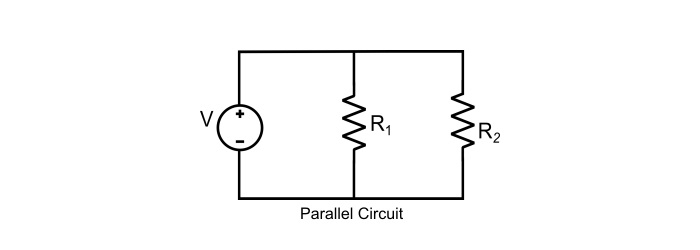• Series–Parallel Circuit − An electric circuit in which some of the circuit components are connected in the series and some of them in parallel, the circuit is known as series-parallel circuit.